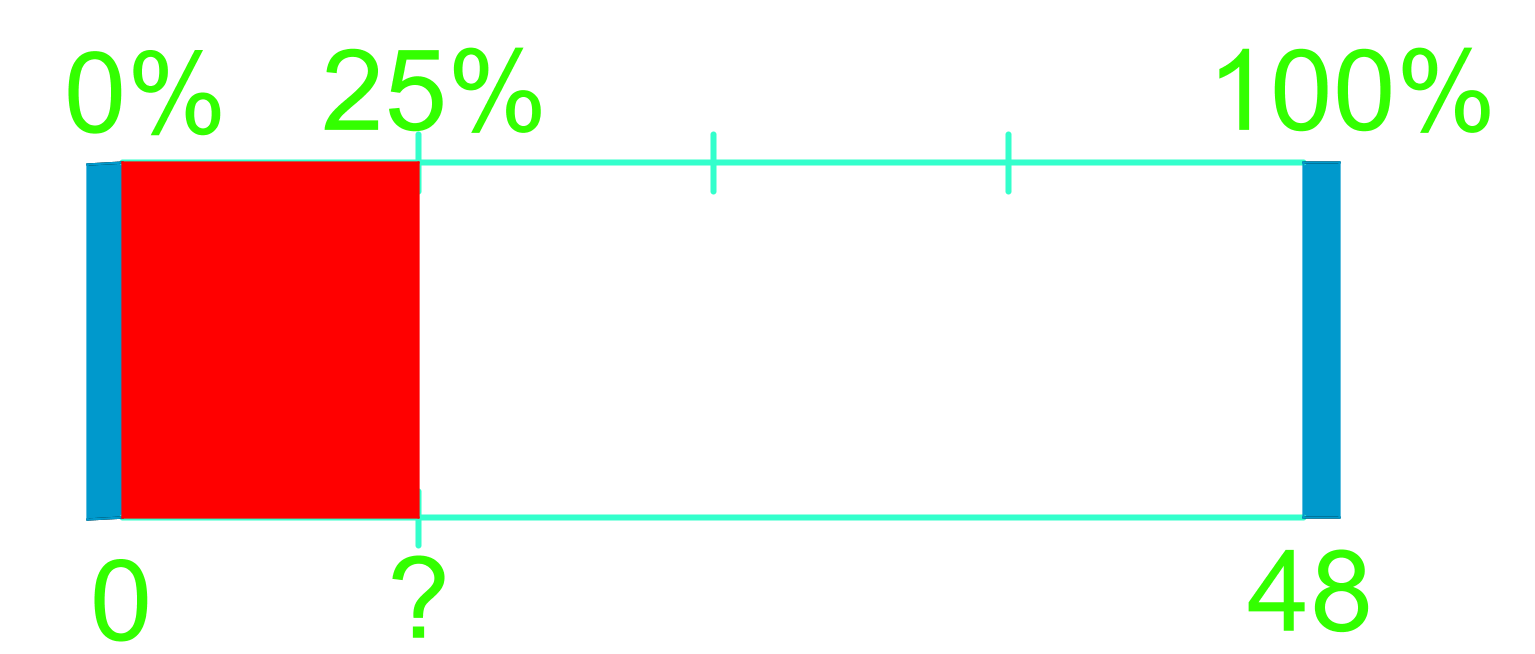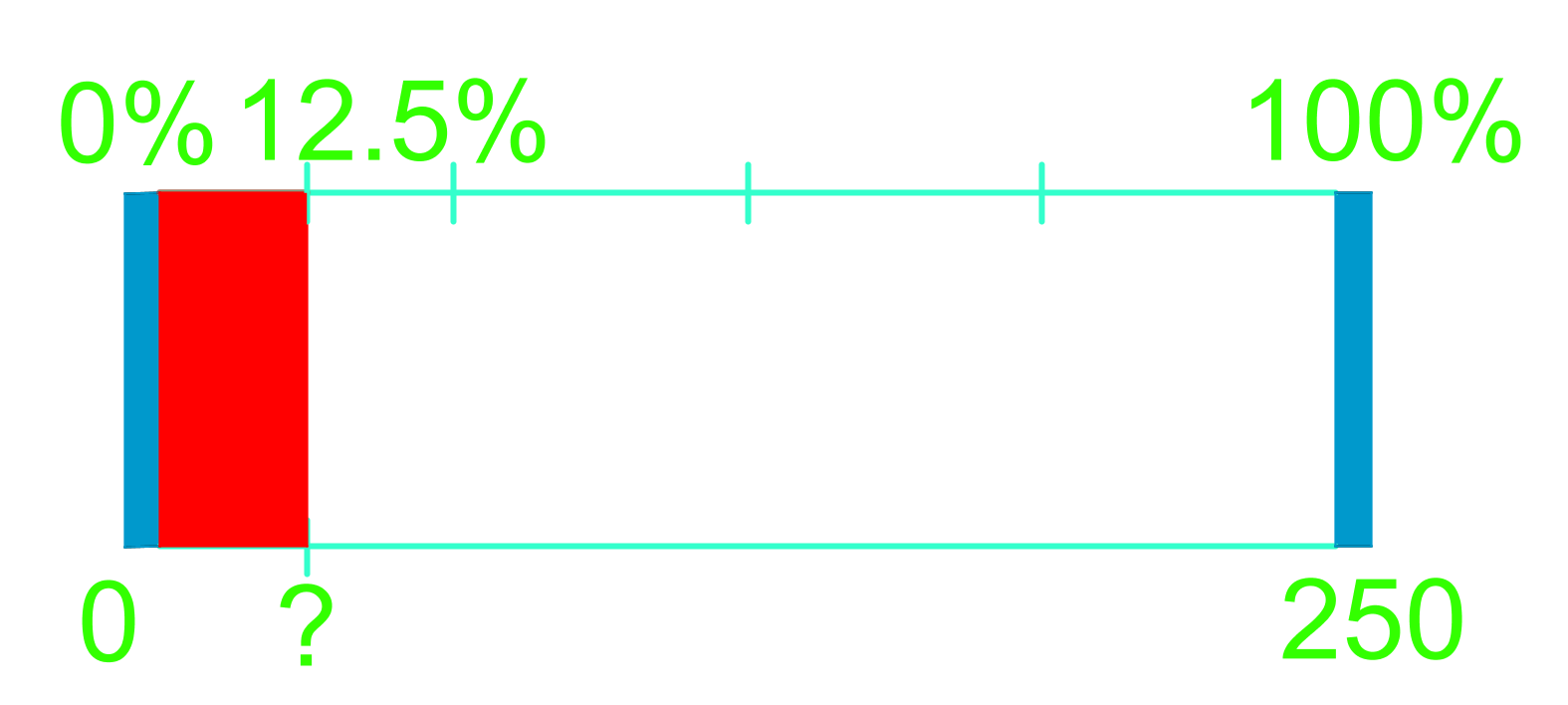# Representing percents

### Representing percents

In this section, we calculate the percents of numbers using loading-strip models. Along with these models, we calculate the percents of numbers using our knowledge from previous sections on adding and subtracting decimal numbers and dividing decimal numbers. For example, to determine 75% of a number, we add the decimal values of 50% and 25% of the number. Also, to determine 50% of a decimal number, we divide that decimal number by 2.

#### Lessons

• 1.
Use the loading-strip model to calculate.
a)
Calculate 25% of 48.b)
Calculate 12.5% of 250.• 2.
Calculate using loading-strip models.
a)
50% of 16 marbles

b)
75% of 30 pink flamingos

c)
10% of 100 Oompa Loompas